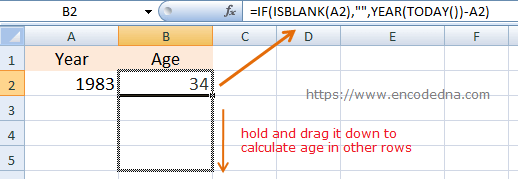﻿ How to Calculate Age in Excel using Just Year

# How to Calculate Age in Excel using Just Year

There are different ways to calculate a person’s age in Excel using different types of data. In most cases you will be given a Date from which you’ll need to get or calculate the age. Here in this post however, I’ll show you the quickest way to calculate the age in Excel using just the Year.Let us assume, I need to input a Year in every row of the first column. Excel should automatically calculate the age and show it in the next column. The above image has the answer though.

Here’s what you can do. In the second column, add this formula.

=IF(ISBLANK(A2),"",YEAR(TODAY()) -A2)

Now enter the year in the first column, the second column will show the age.

To calculate age in other rows, click and hold the second column (that has the formula) using the mouse and drag it down. You can also copy the formula and paste it other rows (where you want to calculate the age).

## What this Formula Does?

The IF function in the formula, is optional. It only checks if the first cell has any value, which it can calculate. If there is no value, it will add blank.

The functions which actually calculates the age are function YEAR() and function TODAY(). It’s a very simple maths. I am subtracting the Current Year with the Year in the first column.

YEAR(TODAY())-A2

The function TODAY() will return today’s date in mm/dd/yyyy format. The YEAR() function, when used with TODAY() will return the current year.